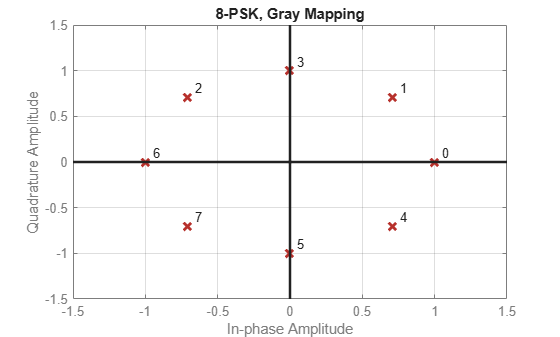pskmod

Phase shift keying modulation

Description

y = pskmod(x,M) modulates the input signal, x, using phase shift keying (PSK) with modulation order M.

example

y = pskmod(x,M,ini_phase) specifies the initial phase of the PSK-modulated signal.

y = pskmod(x,M,ini_phase,symorder) specifies the symbol order of the PSK-modulated signal.

Examples

collapse all

Modulate and plot the constellations of QPSK and 16-PSK signals.

QPSK

Set the modulation order to 4.

M = 4;

Generate random data symbols.

data = randi([0 M-1],1000,1);

Modulate the data symbols.

txSig = pskmod(data,M,pi/M);

Pass the signal through white noise and plot its constellation.

rxSig = awgn(txSig,20);
scatterplot(rxSig)16-PSK

Change the modulation order from 4 to 16.

M = 16;

Generate random data symbols.

data = randi([0 M-1],1000,1);

Modulate the data symbols.

txSig = pskmod(data,M,pi/M);

Pass the signal through white noise and plot its constellation.

rxSig = awgn(txSig,20);
scatterplot(rxSig)Generate random symbols.

dataIn = randi([0 3],1000,1);

QPSK modulate the data.

txSig = pskmod(dataIn,4,pi/4);

Pass the signal through an AWGN channel.

rxSig = awgn(txSig,10);

Demodulate the received signal and compute the number of symbol errors.

dataOut = pskdemod(rxSig,4,pi/4);
numErrs = symerr(dataIn,dataOut)
numErrs = 2

Plot PSK symbol mapping for Gray and natural binary encoded data.

Set the modulation order, and then create a data sequence containing a complete set of constellation points.

M = 8;
data = (0:M-1);
phz = 0;

Modulate and demodulate the data using Gray and natural binary encoded data.

symgray = pskmod(data,M,phz,'gray');
mapgray = pskdemod(symgray,M,phz,'gray');

symbin = pskmod(data,M,phz,'bin');
mapbin = pskdemod(symbin,M,phz,'bin');

Plot the constellation points using one of the symbol sets. For each constellation point, assign a label indicating the Gray and natural binary values for each symbol.

• For Gray binary symbol mapping, adjacent constellation points differ by a single binary bit and are not numerically sequential.

• For natural binary symbol mapping, adjacent constellation points follow the natural binary encoding and are sequential.

scatterplot(symgray,1,0,'b*');
for k = 1:M
text(real(symgray(k))-0.2,imag(symgray(k))+.15,...
dec2base(mapgray(k),2,4));
text(real(symgray(k))-0.2,imag(symgray(k))+.3,...
num2str(mapgray(k)));

text(real(symbin(k))-0.2,imag(symbin(k))-.15,...
dec2base(mapbin(k),2,4),'Color',[1 0 0]);
text(real(symbin(k))-0.2,imag(symbin(k))-.3,...
num2str(mapbin(k)),'Color',[1 0 0]);
end
axis([-2 2 -2 2])Input Arguments

collapse all

Input signal, specified as a vector or matrix of positive integers. The elements of x must have values in the range of [0, M – 1].

Example: randi([0 3],100,1)

Data Types: double

Modulation order, specified as an integer power of two.

Example: 2 | 4 | 16

Data Types: double

Initial phase of the PSK modulation, specified in radians as a real scalar.

If you specify ini_phase as empty, then pskmod uses an initial phase of 0.

Example: pi/4

Data Types: double

Symbol order, specified as 'bin' or 'gray'. This argument specifies how the function assigns binary vectors to corresponding integers.

• If symorder is 'bin', the function uses a natural binary-coded ordering.

• If symorder is 'gray', the function uses a Gray-coded ordering.

Data Types: char

Output Arguments

collapse all

Complex baseband representation of a PSK-modulated signal, returned as vector or matrix of complex values. The columns of y represent independent channels.